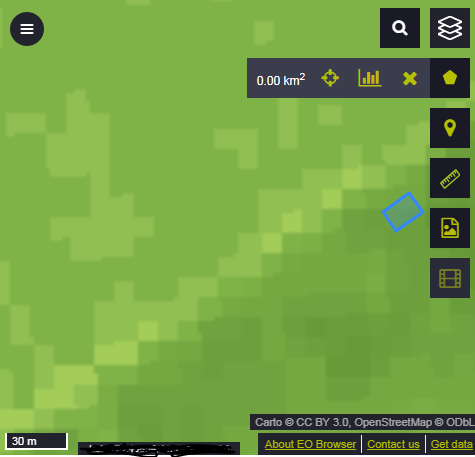# Number of pixels used in a region

I am interesting in getting the NDVI values inside a region. I was able to do a request using FIS as explained here
But I would like to know how many pixels are being used inside the region I defined, for the statistical calculation. Is that posible? how?

Regards

You can add “bins=5” parameter and count them altogether. The minimum number of bins to be used is 4.

I count them using ‘bins=5’ and the total is not an integer (total=170.828125 pixels). Does it means that my region has almost 171 pixels? why is not an integer?

Another related question:
what if I mark a “point of interest” in the eo-browser? How and which pixels are used in that case? I see squares in the layer, and I thought it was a single pixel, but I can mark the “point of interest” in different places inside each square and it gives different statistical values. Also, in the graph, it says “mean”, but…the mean of what values?

Yes, the above is 171 pixels. The “not integer” might be due to your AOI not intersecting exactly with pixels. Or simply due to statistical approximations.

EO Browser takes, if I am not mistaken, an area of 10x10 meters if you choose point option. So by clicking on various parts of the pixel, you will take also some of the neighborhood pixels into account.

The region I was studing is the blue square in the picture. Its area is 192 square meters, If the resolution of the data is 10 m, it should be around 19 pixels, not 171 pixels isn’t it?

regards

There is no picture attached so it is difficult to know for sure. Can you post a request here (hide the instance id)?Thanks, but we will need request URL as well, otherwise it is impossible to analyze what is happening.

Sorry, here is my request:

http://services.sentinel-hub.com/ogc/fis/<INSTANCE_ID>?LAYER=MICAPA&STYLE=INDEX&TIME=2018-08-01%2F2018-08-31&CRS=EPSG:4326&ZOOM=19&GEOMETRY=POLYGON((+37.24932535056907±5.796558136431953,+37.2492447820994±5.7967000985731865,+37.24916350796783±5.796627648970578,+37.24924511126604±5.796486813126811,+37.24932535056907±5.796558136431953))&RESOLUTION=1m&BINS=5

In MICAPA layer I did:

var ndvi = (B08-B04)/(B08+B04);
return [ndvi];

Regards,

rAfA

By defining RESOLUTION=1m, you query interpolated image. If you want to query original data, set RESOLUTION=10m